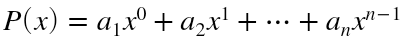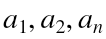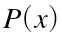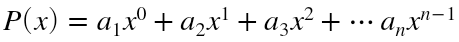# Choose my k-term from matrix and defines by itself on for loop

1 ビュー (過去 30 日間)
Fahmy Shandy 2019 年 12 月 7 日
Commented: Image Analyst 2019 年 12 月 7 日
Suppose i have n×1 matrix before, (column vector), namely "x1" (i'm defining this matrix with x1). I can't write my x1 because of it depends on my input. And this matrix could be 3×1, 7×1, until n×1. In this case i won't mention it on my script below
x1
for c=1:10
for d=0:9
'a',c,=x1(c,1)
end
end
My wish is when i have x1 is matrix of 10×1 (Look at my c) my for loop will display
a1=
2
a2=
3
.
.
.
a10=
4
2, 3, 4 are arbitrary numbers depend on my input of matrix x1. That means, i want it defines a variable by itself generating from my "c" (Look at my fourth line)
And that 'a',c, can be used for evaluating another formula.
For example
Px=a1+a2+a3
Is that possible? Thanks. If my question is not clear, please tell me.
EDIT :
Another alternative in my thought is the following: For example, i want to construct summationMy attempt is:
x1
for c=1:10
for d=0:9
P=sum((x1(c,1)))*9^d
end
end
But it's Not work and displays weird thing.
As i said before, actually my goal is evaluating the k-element in my matrix that is i take it randomly (not literally "random") And construct it as like this:Whereis the k-element from my matrix, and x is just independent variable in that equation and depend on my input.
I've edit my question. I hope it's clear now and tell me if it's not. Thanks again.

#### 0 件のコメント

サインイン to comment.

### 採用された回答

Image Analyst 2019 年 12 月 7 日
It's not clear. Not sure what x1, a, and c are. It looks like the user enters some number of values for x1, then somehow, some way (unknown to me), a vector "a" is generated from x1 because you say the "a" are "arbitrary numbers depend on my input of matrix x1" so it might possibly have values of [2,3,....10] (for one example of "a" that you gave).
Anyway, if you have a vector "a" and another vector x1, you can construct the terms of the series like
theTerms = a .* x1; % Note: a and x1 are either both row vectors, or both column vectors.
so theTerms(1) is the first a times teh first x1, and theTerms(2) is the second a times the second x1, and so on.
So now you can get P(x) like this
Px = cumsum(theTerms);
where Px(1) is theTerms(1), and
Px(2) = theTerms(1) + theTerms(2), and
Px(3) = theTerms(1) + theTerms(2) + theTerms(3), and so on.

#### 2 件のコメント

Fahmy Shandy 2019 年 12 月 7 日
I'm sorry not being clear. a is the element of a column vector x1, and i want randomly choose a for matrix x1, and evaluating it on another formula which is a summation containing a as a constant (notice that a is an element of matrix x1), and x is an independent variable inthat is can be input any numbers that i want.
c is the number of element in matrix x1. For example if i have x1 is a matrix of 10×1, then my c is 10.
What i've done so far
for c=1:n
for d=0:m
P(x)=cumsum((x1(c,1))*x^d)
end
end
Where n and m is some large number depend on my input.
My P(x) isAnd i'm confuse with "cumsum", because my x is a scalar instead of a matrix.
Image Analyst 2019 年 12 月 7 日
Don't use for loops. And you don't need a second index, 1, on x1.
Just do this
x1 = [2, 3, 4, 5, 10] % Whatever
coefficients = 1 : length(x1) % 1, 2, 3, 4, etc.
theTerms = coefficients .* x1; % Note: a and x1 are either both row vectors, or both column vectors.
Px = cumsum(theTerms)
You'll see:
x1 =
2 3 4 5 10
coefficients =
1 2 3 4 5
Px =
2 8 20 40 90

サインイン to comment.